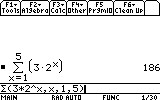# Knowledge Base

## Solution 18469: Calculating the Summation of a Geometric Sequence Using the TI-89 Family, TI-92 Family, or Voyage 200™.

### How can I find the summation of a geometric sequence using a TI-89 family, TI-92 family, or Voyage 200?

The å( ) or summation function can be used to calculate the sum of any range of consecutive terms in a geometric sequence. The syntax for the å function is given below:

å(expression, variable, low, high)

The expression is evaluated for each value of the variable from low to high, and returns the sum of the results. The following example demonstrates how to sum up the first n terms in a geometric sequence.

Find the first 5 terms in the geometric sequence an = 3 * 2n

1) From the home screen, press [F3]  to select 4:å( sum from the Calculus menu.
2) Input the expression 3*2^x.
3) Press [,] [x] to input the variable.
4) Press [,]  [,]  to input the low and high values.
5) Press [ ) ] [ENTER].The result of the summation displayed should be 186.

Keep in mind that the å( ) function does not allow a step size to be entered; it always uses the default value of 1. However, if a different step size is required (such as for Riemann sums), a combination of both the sum( ) and seq( ) functions can be used in order to do this. Both functions are accessed by pressing [2nd] [MATH]  from the home screen. The syntax for this calculation would be essentially the same, except for the additional optional parameter of step size at the end. The sum(seq( syntax is listed below:

sum(seq(expression, variable, low, high, step)The result of the summation displayed should be 126.

Please see the TI-89 family, TI-92 family, or Voyage 200 guidebooks for additional information.